Subsections

# 14. wavdetect Parameters & Data Products Reference

This chapter lists all the wavdetect parameters in alphabetical order for quick reference. In addition, a guide to the data products output by wavdetect is provided.

# 14.1 Default Parameter File

unix% plist wavdetect

Parameters for /soft/ciao/param/wavdetect.par

#
#   parameter file for wavdetect
#
#
#   input
#
infile =                  Input file name
#
#   output
#
outfile =                  Output source list file name
scellfile =                  Output source cell image file name
imagefile =                  Output reconstructed image file name
defnbkgfile =                  Output normalized background file name
#
#   scales
#
scales = 2.0 4.0          wavelet scales (pixels)
(regfile = )                ASCII regions output file
#
#   output options
#
(clobber = no)              Overwrite existing outputs?
(kernel = default)         Output file format (fits|iraf|default)
(ellsigma = 3.0)             Size of output source ellipses (in sigmas)
(interdir = .)               Directory for intermediate outputs
#
#########################################################################
#
#   wtransform parameters
#
#
#   optional input
#
(bkginput = )                Input background file name
(bkgerrinput = no)              Use bkginput for background error
#
#   output info
#
(outputinfix = )                Output filename infix
#
#   output content options
#
(sigthresh = 1e-06)           Threshold significance for output source pixel list
(bkgsigthresh = 0.001)           Threshold significance when estimating bkgd only
#
#   exposure info
#
(exptime = 0)               Exposure time (if zero, estimate from map itself
(expfile = )                Exposure map file name (blank=none)
(expthresh = 0.1)             Minimum relative exposure needed in pixel to analyze it
#
#   background
#
(bkgtime = 0)               Exposure time for input background file
#
#   iteration info
#
(maxiter = 2)               Maximum number of source-cleansing iterations
(iterstop = 0.0001)          Min frac of pix that must be cleansed to continue
#
#   end of wtransform parameters
#
########################################################################
########################################################################
#
#   wrecon parameters
#
#
#   PSF size parameters
#
(xoffset = INDEF)           Offset of x axis from optical axis
(yoffset = INDEF)           Offset of y axis from optical axis
(eband = 1.4967)          Energy band
(eenergy = 0.393)           Encircled energy of PSF
(psftable = \${ASCDS_CALIB}/psfsize20010416.fits -> /soft/ciao/data/psfsize20010416.fits) Table of PSF size data
#
#   end of wrecon parameters
#
########################################################################
#
#   run log verbosity and content
#
(log = no)              Make a log file?
(verbose = 0)               Log verbosity
#
#   mode
#
(mode = ql)


# 14.2 Parameter Descriptions

In the following parameter descriptions, "required=yes" indicates that the user must provide a value for the indicated parameter (e.g. infile) before the program will run. Also, the empty string (" ") is equivalent to the string none for filenames.

• bkgerrinput
Use bkginput for background error
type=boolean
def=no
required=no

Whether the background error data in the bkginput file will be used.

If bkgerrinput is changed to yes, then the background error data in the second extension of the bkginput file will be used (i.e. "bkginputfilename.fits'').

• bkginput
Input background file name
type=string
filetype=input
required=no

The name of a background image.

If desired, a previously computed background image may be input instead of allowing wavdetect to construct a default normalized background. When using bkginput , enter none for the default normalized background output file (defnbkgfile ).

• bkgsigthresh
Threshold significance when estimating bkgd only
type=real
def=0.001
required=no

The significance threshold for cleansing data from the image to compute the background map.

As it does not effect source detection, this parameter should be set to a more liberal value than sigthresh (e.g. 10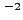or 10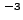, the default), but not smaller than sigthresh ). This will help reduce the effect of weak undetectable sources on the background map calculation. This value should be no larger than 0.05, the usual 5% (or 95%) statistical criterion for rejecting the null hypothesis, which is that the pixel in question has data sampled solely from the background.

• bkgtime
Exposure time for input background file
type=real
def=0
required=no

Exposure time for the background file.

The bkgtime parameter may specify the LIVETIME value for the provided background (bkginput ). If bkgtime is non-zero and no background map is supplied, the source characteristics in the output file will be wrong.

• clobber
Overwrite existing outputs?
type=boolean
def=no
required=no

If "yes", overwrite existing outputs.

wavdetect does not not overwrite existing outputs unless clobber is set to yes.

• defnbkgfile
Output normalized background file name
type=string
filetype=output
required=yes
autoname=yes

Output default normalized background output file.

The output file that will contain the default normalized (i.e. flat-fielded) background is specified by the defnbkgfile parameter. During its second stage, wavdetect makes one normalized background estimate from the stack of per-scale normalized backgrounds produced during the first stage. It writes the computed background to this file. If autonaming is used (i.e. "defnbkgfile=."), then the output file will be named "<infile_root>_nbkg".

• eband
Energy band
type=real
def=1.4967
units=keV

The photon energy (keV) at which the point spread function (PSF) is chosen.

The selection of a particular PSF size is governed by the energy band eband parameter [keV], since the Chandra PSF is a function of energy. By default the energy band is set to 1.4967 keV.

• eenergy
Encircled energy of PSF
type=real
def=0.393
required=no

The percentage of PSF energy to be encircled, expressed as a fraction of 1.0.

wavdetect also uses an encircled energy percentage value, given by the eenergy parameter, in concert with the calibration PSF file. This value is the percentage of PSF energy to be encircled by the detect cell, expressed as a fraction of 1.0. The eenergy is a key parameter for detecting off-axis sources, and users are urged to experiment with other values. By default, the value is set to 0.393 (the 1-sigma integrated volume of a normalized two-dimensional Gaussian); another suggested value to try is 0.5. Note that the efficiency of the algorithm is reduced if this fraction is too high or too low. If the value of eenergy is set too high (e.g. 0.9), there is the risk that the computed source cell will contain more than one source, rendering source property estimates moot. On the other hand, if the value is set too low, then the computed cell may contain only a fraction of the source counts.

• ellsigma
Size of output source ellipses (in sigmas)
type=real
def=3.0

The size, in sigmas, to make the elliptical source detection regions.

The size of the elliptical source regions in both of the source region files (regfile and outfile ) is controlled via the ellsigma parameter. This parameter is a multiplicative factor applied to sigma, the standard deviation of the distribution, to scale the major and minor axes of the ellipses for each source detection.

The distribution is defined as the distribution of counts within the source cell (i.e. the observed counts), and is assumed to be Gaussian. Thus, if ellsigma is 1, then the elliptical source region will contain 39.3% of the source counts (i.e. the 1-sigma point for a 2-D Gaussian).

This feature is included so that the graphics overlay may be made more visible; it is does not affect the detections themselves, only the display of the regions.

• expfile
Exposure map file name (blank=none)
type=string
filetype=input

The name of an exposure map file.

This is an image of the data set exposure time. Although the expectation is that expfile will have units of time, exposure maps generated by the CIAO tool mkexpmap, which have units of cm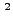, work as well.

• expthresh
Minimum relative exposure needed in pixel to analyze it
type=real
def=0.1
required=no

The minimum relative exposure needed in a pixel in order to analyze it.

For each pixel, a relative exposure (pixel exposure value over maximum value of exposure in map) is calculated. If this relative exposure is less than the parameter expthresh , then the pixel in question is not analyzed; all associated values (background, correlation, etc. ) are set to zero.

This parameter should not be less than 0.1 or the accuracy of normalization will be too low. If set close to 1, only very limited regions of the field of view will be considered when source correlation maxima are listed. Typical values are 0.1-0.2.

• exptime
Exposure time (if zero, estimate from map itself
type=real
def=0
units=seconds

The length of time over which the field was observed, in seconds.

If set to 0, the value will either be taken from the expfile FITS header or, failing that, estimated by averaging over exposure map pixel values at the center of the field.

• imagefile
Output reconstructed image file name
type=string
filetype=output
required=yes
autoname=yes

The name of a reconstructed source image output file.

The reconstructed source image filename is given by the imagefile parameter. If autonaming is used (i.e. "imagefile=."), then the output file will be named "<infile_root>_image".

• infile
Input file name
type=string
filetype=input
required=yes

The name of the input file.

The input file can an event list or an image. CIAO Data Manipulation (DM) syntax may be used in the input file specification, as explained in Chapter 1, Section 1.3.3.

Be careful using images larger than 1024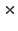1024, as a large amount of computer memory must be available.

Pixel values should be reasonable numbers, as /wavdetect/ probability distributions have been derived assuming Poisson statistics. /wavdetect/ simply will not work with fluxed images, as the calculated correlation values are so small that no detections occur.

• interdir
Directory for intermediate outputs
type=string
filetype=output
def=.
required=no

A directory for intermediate results.

• iterstop
Min frac of pix that must be cleansed to continue
type=real
def=0.0001
required=no

The minimum fraction of pixels that must be cleansed to continue constructing the background.

If the ratio of newly cleansed pixels to the overall number of pixels in the dataset is less than the parameter iterstop when wavdetect constructs a default normalized (i.e. flat-fielded) background (defnbkgfile ), then wavdetect stops iterating and uses the current background estimate as the final background estimate. The background is calculated if there are very few new pixels being cleansed (see bkgsigthresh ).

The iterstop parameter specifies how many iterations the program should go through to calculate the background. A typical value is 0.0001 (the default): stop iterating when one of every thousand pixels has been cleansed. This parameter should not be smaller than one over the number of pixels in the dataset or larger than 1.

• kernel
Output file format (fits|iraf|default)
type=string
def=default
required=no

The format for the output file.

The kernel parameter controls the output format (default, fits, or iraf); the default is for the output file to have the same format as the input file.

• log
type=boolean
def=no
required=no

The verbosity of log information is sent to STDOUT (usually the screen). However, if log is set to yes, then the files wrecon.log and wtransform.log will be created.

• maxiter
Maximum number of source-cleansing iterations
type=integer
def=2
required=no

The maximum number of iterations per scale pair for cleaning sources from the data in order to estimate the background map.

Increasing this number will increase the method's source detection sensitivity, but may not increase it enough to justify the increased computation time. More iterations are generally needed for large wavelet scales (e.g. 16 pixels in a Rosat 512512 PSPC field). Note that the tool may converge before reaching maxiter .

• mode
def=ql

The mode defines how to handle querying for parameters. For example, setting the mode to "h'' means that no prompting will occur; this is useful when running the tool from a script. See "ahelp parameter'' for more information and a list of all the available modes.

• outfile
Output source list file name
type=string
filetype=output
required=yes
autoname=yes

The name of the output file.

The outfile parameter is used to provide a name for the output file. The kernel parameter controls the output format; the default is for the output file to have the same format as the input file. If autonaming is used (i.e. "outfile=."), then the output file will be named "<infile_root>_src".

• outputinfix
Output filename infix
type=string
required=no

A unique string for use in output filenames.

This may be used to avoid file naming collisions with other wavdetect users.

• psftable
type=string
filetype=ARD
def=psfsize20010416.fits

The path and name of the file containing PSF information.

In wavdetect , PSF, energy, and offset information supplied via these parameters is used to select a source counts image; a source cell is computed using this image, and source properties are estimated using the data within the computed cell. Note that, unlike celldetect , these parameter values have no effect on the detection process. Instead, these parameter values affect reported source properties.

The PSF information used by wavdetect is contained in a Chandra calibration file distributed with CIAO , whose location is given by the value of the psftable parameter; by default this parameter is automatically set to point to the appropriate Chandra calibration PSF file.

• regfile
ASCII regions output file
type=string
autoname=yes

The name of an ASCII source region output file.

In addition to the output source list (outfile ), the user may choose to have an ASCII source region file written by specifying a filename in regfile parameter. If autonaming is used (i.e. "regfile=."), then the source regions output file will be named "<infile_root>_reg".

This region file contains the location and size of the principal axes, for each detected source elliptical region. The elliptical region size is such that the region contains some fraction of the source counts. The parameter ellsigma specifies the desired source count fraction.

• scales
Wavelet scales (pixels)
type=string
def=2.0 4.0

The scales parameter determines how many scaled transforms will be computed. wtransform produces a complete set of outputs for each scale. Small scales tend to detect small features, and larger scales find larger features. The units for scales are pixels. The value of scales is the radius of the Mexican Hat function, which crosses zero at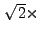radius.

For the initial run, try the default value "2.0 4.0". If that test is successful, try again with scales "1.0 2.0 4.0 8.0 16.0". For a more extensive run, the user might wish to try the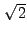series: "1.0 1.414 2.0 2.828 4.0 5.657 8.0 11.314 16.0".

The primary concern regarding this parameter is to match the first scale size to the point spread function (PSF). Once that is done, the user must decide how many scaled wavelets to use. To some extent, choices are based on the computing resources at hand. Reasonable inputs are a 512512 array and 5 to 9 scales, where each scale is a factor of 2 orlarger than the preceeding one.

In practice, one must not have too many pixels or scale sizes, otherwise the computational load becomes a problem. Data structures for a 512512 image use up 36 MB, while a 20482048 image requires over 300 MB. Datasets that do not fit in physical memory will page heavily to disk and processing will progress very slowly. Scale sizes larger than 32 allocate excessive memory because it is necessary to pad the image with surrounding zeros.

To deal with resolved sources, several convolutions are performed, each with a successively larger scale version of the wavelet. The resulting "correlation maps" are then examined for regions where the intensity is larger than some threshold, and the final source list is constructed from a comparison of the different scale runs.

• scellfile
Output source cell image file name
type=string
filetype=output
required=yes
autoname=yes

The output image of the source cells.

The scellfile is the image showing the source cells. These source cells delimit the image pixels that are used to estimate source properties (e.g. count rate). If autonaming is used (i.e. "scellfile=."), then the output file will be named "<infile_root>_scell".

• sigthresh
Threshold significance for output source pixel list
type=real
def=1e-06
required=no

The significance threshold for identifying a pixel as belonging to a source.

A good value to use is the inverse of the total number of pixels in the image, e.g.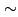10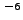(the default) for a 10241024 field. This is equivalent to stating that the expected number of false sources per field is one. If larger arrays are used without decreasing the value of sigthresh , there will be an increased probability of detecting false sources. Likewise, if smaller arrays are used, the user may wish to increase this parameter value. The sigthresh should not be smaller than roughly10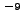to10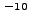; at this value, the accuracy of the computed detection thresholds is unknown.

• verbose
Log verbosity
type=integer
def=0
min=0
max=5
required=no

The verbosity of log output.

The verbosity of log information ranges from 0 (none) to 5 (maximum output) and is sent to STDOUT (usually the screen).

• xoffset
Offset of x axis from optical axis
type=integer
def=INDEF
units=pixels
required=no

The offsets of the x-axis for the calculation of the off-axis angle.

By default, celldetect calculates the off-axis angle using the nominal pointing of the data file as the origin. Users may change this behavior by setting offsets to any numerical values (using the xoffset and yoffset parameters); these offsets provide the location of the optical axis with respect to the center of the data file. A typical scenario in which the user may select to use offsets is when the data file is a sum of two or more observations with different pointings. The nominal pointing in such a data file is usually poorly defined and the off-axis angle could be calculated from an undesired origin, leading to suboptimal selection of the detect cell size.

• yoffset
Offset of y axis from optical axis
type=integer
def=INDEF
units=pixels
required=no

The offsets of the y-axis for the calculation of the off-axis angle.

See the description for xoffset .

# 14.3 Data Product Descriptions

Source List File (outfile )

The primary output of wavdetect is the FITS file of source detections. The following properties are recorded in the output file:

 Data item Unit Description RA deg Source right ascension DEC deg Source declination RA_ERR deg Source right ascension error DEC_ERR deg Source declination error POS(X,Y) pixel Physical coordinates X_ERR pixel Source X position error Y_ERR pixel Source Y position error NPIXSOU pixel Pixels in source region NET_COUNTS count Net source counts NET_COUNTS_ERR count Error in net source counts BKG_COUNTS count Background counts (scaled to source cell) BKG_COUNTS_ERR count Error in background counts NET_RATE Source count rate (count/exposure map units) NET_RATE_ERR Source count rate error BKG_RATE Background count rate (count/exposure map unit) BKG_RATE_ERR Background count rate error EXPTIME Effective exposure map value (exposure map unit) EXPTIME_ERR Effective exposure map error SRC_SIGNIFICANCE Source significance PSF_SIZE pixel Estimated size of PSF MULTI_CORREL_MAX 1 if source contains 2+ correl maxima SHAPE Shape of source region R Radii of source region ROTANG deg Rotation angle of source region PSFRATIO The ratio of the equivalent sigma of the count distribution to the PSF_SIZE COMPONENT Source number

Source cells are arbitrarily shaped groups of pixels within which source properties are estimated. A given source's cell is constructed by: (1) estimating the PSF size; (2) choosing the source counts image created using a smoothing scale closest to that PSF size; and (3) going to the source's location in that image, where the image amplitude is positive, and determining the closest zero-amplitude contour around that point. (If there is a saddle point in the source counts distribution closer than the zero-point in a particular direction, then the saddle point is used to define the contour.) Pixels within that contour constitute the source cell.

• RA, DEC:
Right ascension and declination coordinates, corresponding to X and Y. Calculated only if WCS keywords are present in the data, otherwise filled with zeros.

• RA_ERR, DEC_ERR:
RA and DEC errors, corresponding to X_ERR and Y_ERR.

• POS(X,Y):
Estimated X and Y positions of the detected source. Calculated with a "center-of-mass" algorithm using the counts data within the source cell. If the cell is asymmetric and/or the source is relatively weak, the position may be offset from the true position apparent to the eye.

• X_ERR, Y_ERR:
Estimated uncertainties on the X or Y position of the source.

• NPIXSOU:
The number of image pixels contained in the source cell.

• NET_COUNTS:
The sum of all counts in the source cell minus the sum of the estimated background counts.

• NET_COUNTS_ERR:
Estimated uncertainty of NET_COUNTS. This estimate does not include contributions from covariance terms (which are non-zero because the background estimates in adjacent pixels are not statistically independent); these contributions are generally ignorable.

• BKG_COUNTS:
The sum of the estimated background counts in each pixel in the source cell. The estimated background is output as the default normalized background image.

• BKG_COUNTS_ERR:
Estimated uncertainty of BKG_COUNTS. This estimate does not include contributions from covariance terms (which are non-zero because the background estimates in adjacent pixels are not statistically independent); these contributions are generally ignorable.

• NET_RATE:
Source count rate: the NET_COUNTS divided by EXPTIME. Note that the units of NET_RATE are not necessarily seconds: typical Chandra exposure maps have units cm, so the NET_RATE is in counts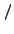cm.

• NET_RATE_ERR:
Estimated uncertainty of NET_RATE. This estimate does not include contributions from covariance terms (which are non-zero because the background estimates in adjacent pixels are not statistically independent); these contributions are generally ignorable.

• BKG_RATE:
Background count rate: the BKG_COUNTS divided by EXPTIME. Note that the units of BKG_RATE are not necessarily seconds: typical Chandra exposure maps have units cm, so the BKG_RATE is in countscm.

• BKG_RATE_ERR:
Estimated uncertainty of BKG_RATE. This estimate does not include contributions from covariance terms (which are non-zero because the background estimates in adjacent pixels are not statistically independent); these contributions are generally ignorable.

• EXPTIME:
The sum of the exposure within the source cell. Note that the units of EXPTIME are not necessarily seconds: typical Chandra exposure maps have units cm.

• EXPTIME_ERR:
Estimated uncertainty of EXPTIME. This estimate takes into account the Poisson fluctuations of the data within each pixel of the source cell, but ignores any uncertainty in the pixel-by-pixel estimate of EXPTIME itself.

• SRC_SIGNIFICANCE:
A significance estimate in units of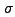, found by dividing the NET_COUNTS by the "Gehrels error"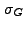of the BKG_COUNTS (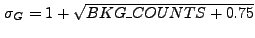). This significance should not be taken at face value, particularly in the low-counts limit. The best statement of a source significance, regardless of the value of SRC_SIGNIFICANCE, is that it is less than or equal to the input value sigthresh .

• PSF_SIZE:
The estimate "size," in image pixels, of the PSF at the source location. This is an encircled-energy radius, not a diameter.

• MULTI_CORREL_MAX:
If 1, then multiple correlation maxima are observed within the output source cell: an indication that a detected source may in actuality be a composite of more than one source.

• SHAPE:
The shape of region output to the ASCII source region file; ellipse by default.

• R:
The estimated semi-major and semi-minor axis lengths (i.e. the x and y radius lengths in pixels, before rotation). For SHAPE = ellipse, this is ellsigma times the axis lengths.

• ROTANG:
The counterclockwise angle between the semi-minor axis (see R) and the y-axis of the image, in degrees.

• PSFRATIO:
The ratio of the estimated "radius" of the source cell to PSF_SIZE. The radius of the source cell is found by taking the square root of the product of the two components of R and dividing this by 2*ellsigma. A ratio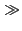1 is an indication that the detected source may be extended.

• COMPONENT:
The number assigned to the detected source. The source numbers correspond to those in the output source list. Its primary use is for filtering of the source list by CIAO tools (e.g. "component=1:10", "component=1,2,5").

ASCII Source Region File (regfile )

In addition to the source list file (outfile ), the user may also select to have a source region file (regfile ) output.

This region file is useful for subsequent input into ds9, particularly to overlay region markers over each detection; see Chapter 1, Section 1.4 for details.

The source region file from the example in Chapter 11, Section 11.2.2:

unix% more example2_out.reg
ellipse(251.973913,268.647826,14.517026,8.139225,102.509720)
ellipse(251.878641,309.131068,8.314850,7.646153,26.671946)
ellipse(251.925620,320.471074,8.546168,7.646372,90.888733)
.
.
.


Log File (logfile )

If log is set to yes, then the files wrecon.log and wtransform.log are created.

The log files from the example in Chapter 11, Section 11.2.2:

unix% more wrecon.log
Input image file: data/datasetA_acis_img.fits[123:630,109:614]
Input correlation maxima stack: ./wd_srclist_stk
.
.
.

unix% more wtransform.log
input file: data/datasetA_acis_img.fits[123:630,109:614]
exposure file:
correlation maxima output stack: ./wd_srclist_stk
correlation image output stack: ./wd_correl_stk
.
.
.


Source Cell Image File (scellfile )

The source cell image shows which pixels are used in the computation of properties for each detected source. The value for each pixel is either 0 (not a source pixel) or an integer (corresponding to a particular numbered source in the source list, i.e. a pixel value "2" means that that pixel is associated with source #2). The extent of each source cell is determined by using the particular source counts image closest in size to the PSF size at the source location; see Chapter 13 for more details on /wavdetect/ theory.

Reconstructed Image File (imagefile )

The reconstructed source image is a noise-free reconstruction of the raw data using the correlation images output from wtransform . Note that the overall normalization of the source image differs from that of the raw data image, thus values in particular pixels should not be read as estimates of the number of counts in those pixels. Chapter 13 contains details on how this image is constructed.

Normalized Background File (defnbkgfile )

During its second stage, wavdetect makes one normalized background estimate from the stack of per-scale normalized backgrounds produced during the first stage. It writes this computed background to this file.

The normalized (or flat-field) background image shows the number of expected background counts in each detector pixel, if the exposure time in each pixel is constant and equal to the amount of time the field was observed.

Computationally, the normalized background is: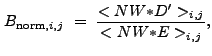(14.1)

where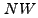represents the wavelet function with positive values reset to zero ("Negative Wavelet"),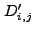are the cleansed data in each pixel (the data with putative source counts removed), and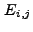is the ratio of exposure time in each pixel to the overall observation time.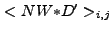and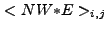are the correlation of the negative wavelet function with the cleansed data and exposure, respectively.

Because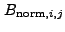is a function of wavelet scale size, there is a distinct normalized background image output from wtransform at each chosen pair of scales (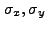).